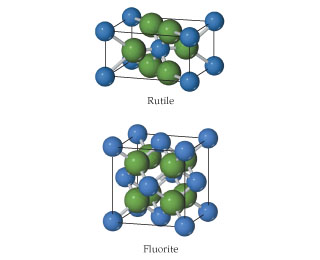# Problem: The rutile and fluorite structures, shown here (anions are colored green and are the larger of the two spheres), are two of the most common structure types for ionic compounds where the cation-to-anion ratio is 1:2.For CaF2 and ZnF2 use ionic radii, Ca2+ (r=1.14), Zn2+ (r=0.88), and F- (r=1.19), to predict which compound is more likely to crystallize with the fluorite structure and which with the rutile structure.

###### FREE Expert Solution

We are going to use the ratios of the ionic radius to identify which structure fits which compound.

• rutile → octahedral → ratio of 0.414 - 0.732
• flourite → cubic → ratio of 0.732 - 1.0

We are going to calculate the ratios using the formula below:

82% (284 ratings)###### Problem Details

The rutile and fluorite structures, shown here (anions are colored green and are the larger of the two spheres), are two of the most common structure types for ionic compounds where the cation-to-anion ratio is 1:2.For CaF2 and ZnF2 use ionic radii, Ca2+ (r=1.14), Zn2+ (r=0.88), and F- (r=1.19), to predict which compound is more likely to crystallize with the fluorite structure and which with the rutile structure.

Frequently Asked Questions

What scientific concept do you need to know in order to solve this problem?

Our tutors have indicated that to solve this problem you will need to apply the Atomic, Ionic, and Molecular Solids concept. If you need more Atomic, Ionic, and Molecular Solids practice, you can also practice Atomic, Ionic, and Molecular Solids practice problems.

What professor is this problem relevant for?

Based on our data, we think this problem is relevant for Professor Qasem's class at NEWARK.